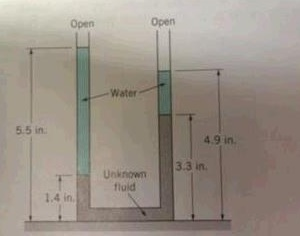# What must be the value of the specific weight of the fluid for the configuration shown in the...

## Question:

What must be the value of the specific weight of the fluid for the configuration shown in the image. Express your answer in {eq}lb/ft^3 {/eq}## Pressure at a Point:

According to Pascal's law, the pressure at a point in a static fluid is equal in magnitude in all directions. Manometers are used to measure the pressure or difference in pressure based on the height of the liquid column, {eq}p= \rho gh {/eq}, where, {eq}\rho {/eq} is the density of the fluid and g is the acceleration due to gravity.

Note: The product of the density of the fluid and the acceleration due to gravity is the specific weight of the fluid.

Step 1

Equate the pressure at the interface of the two fluids in the left limb of the U-tube with the right limb of the U-tube:

{eq}\left(\rho_w...

Become a Study.com member to unlock this answer! Create your account